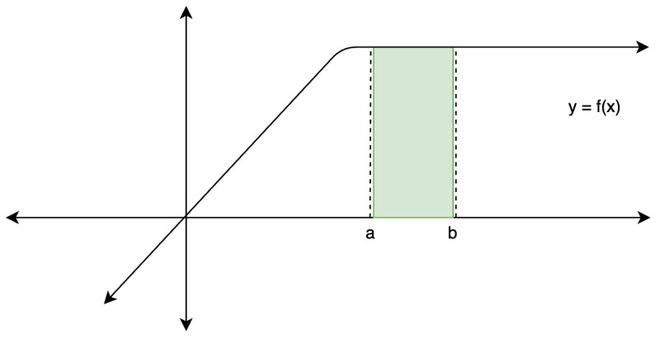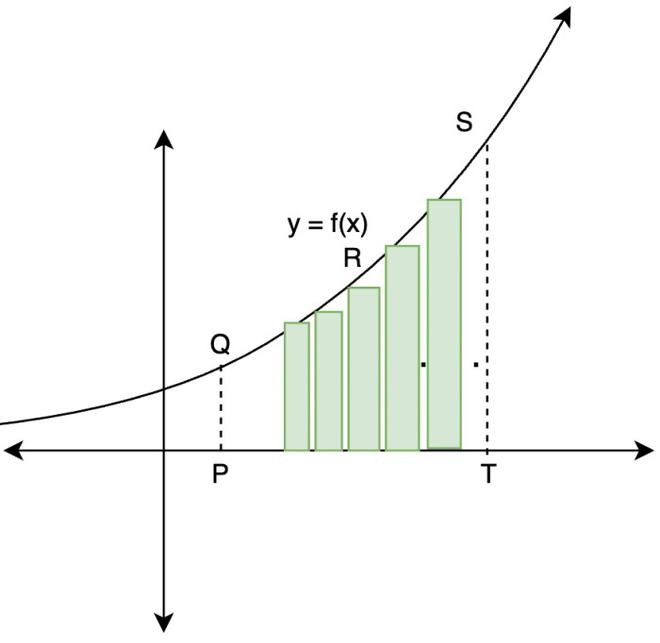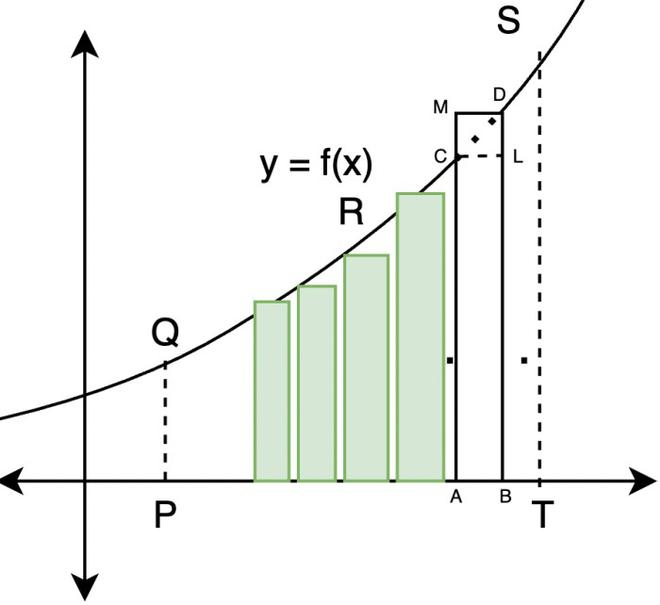Open in App
Not now

## Related Articles

• CBSE Class 12 Syllabus
• CBSE Class 12 Maths Notes
• CBSE Class 12 Physics Notes
• CBSE Class 12 Chemistry Notes
• CBSE Class 12 Accountancy Notes
• CBSE Class 12 Computer Science (Self-Paced Course)

# Definite Integral

• Last Updated : 16 Sep, 2022

Usually, integrals up until now are evaluated as a function or an algebraic expression in terms of the variable. Definite Integrals evaluate up to a constant; they give us a unique value. Intuitively definite integrals are representative of the area under a curve from one position to another position. These positions are declared before evaluating the integral as limits of the integrals.Definite Integral gives the area between these two points

Let’s define the definite integral formally now,

## Definite Integral

Definite integral is denoted by. Here, ‘a’ and ‘b’ are the limits of the integral. “a” is called the upper limit of the integral, and “b” is called the lower limit.

The definite integral can be introduced either as the limit of a sum or if it has an antiderivative in the interval [a, b], then its value is the difference between the values of F at the endpoints, i.e., F(b) – F(a).

## Evaluating Definite Integrals

Finding the area enclosed by a graph of any function within limits mentioned (say, [a, b]) on the graph is known as evaluating a Definite Integral. In Definite Integrals, the limit goes from a to b.It is equal to the area in figure above x-axis and below y-axis.

Question 1: Integrate the definite integral,Solution:

Integrating,Question 2: Evaluate,Solution:## Definite Integral as Limit of a Sum

Assuming that ƒ is a continuous function and positive on the interval [a, b]. So, its graph is above the x-axis.

The definite integralis the area bounded by the curve y = f(x), the ordinates x = a and x = b and x-axis.

To evaluate this area, consider the region PQRSTP in the figure below:Let P be x = a and T be x = b. Divide the interval [a, b] into n equal subintervals denoted by [x0, x1], [x1, x2], [x2, x3] ….[xr-1, xr] …..[xn-1, xn] where x0 = a, x1 = a + h, x2 = a + 2h …. and xn = a + nh or n =. As n ⇢ ∞, h ⇢ 0.

The region PRSQP under consideration is the sum of n subregions, where each subregion is defined on subintervals [xr – 1, xr], r = 1, 2, 3, …, n.It can be seen in the above figure that.

Area of the rectangle (ABLC) < area of the region (ABDCA) < area of the rectangle(ABDM)

As xr– xr–1 → 0, i.e., h → 0, all the three areas shown in the figure become nearly equal to each other. Now we form the following sums,sn and Sn denote the sum of areas of all lower rectangles and upper rectangles raised over subintervals [xr-1, xr] for r = 1, 2, 3,…. respectively.

As n → ∞ strips become narrower and narrower, so, the limiting values of (2) and (3) are the same in both cases and the common limiting value is the required area under the curve.

So,Now, this equation can also be re-written as,Where,This expression is knows as definition of definite integral as limit of sum.

Question 1: Findas the limit of sum.

Solution:

By the definition given above,whereHere, a = 0 and b =2, f(x) = x2 + 1, h =Question 2: IntegrateSolution:Applying G.P, a=1. r=e2/n, we will get,Using,Question 3: EvaluateSolution:

a=-1, b=2, f(x)=5x

h=(b-a)/n=3/n, nh=3

By definition:## Properties of Definite Integral

Property 1: This property states that the limits are interchangeable on definite integrals with an extra negative sign.Property 2: This property has limits from a to itself; therefore, the figure is nothing but a point, and there is no area of a point. Therefore, the integration with such limits is always zero.Property 3: This property is valid since C is a Constant that can be taken out from the integration easily as it is not included in the function given.Property 4: This property states that the value of the Integration will remain the same after splitting the function connected with sum or difference.Property 5: This property helps us solve an integral in adjacent parts. As it is clear that one more limit c is added in RHS where c lies between a and b only.Property 6: This property states that the variable used to integrate the function does not matter as long as the limits and the value of the function are the same.Property 7:Here, C is any constant.

Property 8: This property says that if the value of the function is greater than zero, then its integration will also be greater than zero.

If f(x) ≥ 0 for a ≤ x ≤ b then≥ 0.

Property 9: If the value of a function is greater than the value of another function, then the integration of that function will also be greater than the integration of the other function.

If f(x) ≥ g(x) for a ≤ x ≤ b thenProperty 10: If p ≤ f(x) ≤ P for a≤x≤b then p[a-b]≤≤ P[a-b]

Property 11:Property 12:## Fundamental Theorems of Calculus

We have defined definite integral as area enclosed by function f(x) from x = a to x = b. So, the definite integral is also called the area function. We denote this area function by A(x), it is given by,Based on this definition, we will state two fundamental theorems.

First Fundamental theorems of Calculus:

Let f be a continuous function on the closed interval [a, b], and let A (x) be the area function. Then A′(x) = f (x), for all x ∈ [a, b].

Second Fundamental Theorem of Calculus:

Let f be a continuous function defined on the closed interval [a, b] and F be an antiderivative of f. ThenNote: This theorem is really useful as it gives us means of calculating a definite integral without actually calculating it a limit of a sum

### Steps for Calculating

• Find the indefinite integral. Let’s call it F(x). There is no need to keep constant “C”, it will cancel out anyway in the end.
• Just find F(b) – F(a) = [F(x)]ba which is the value of this definite integral.

Let’s look at an example of this theorem.

Question 1: Calculate the integral:Solution:

It can be solved using the second fundamental theorem of the calculus.For finding the definite integral, let’s plug in the values. Let the value of definite integral be S. and a= 2 and b = 3.Question 2: Find the Integral:Solution:

Solving,Question 3: EvaluateSolution:2log2-2+1=(2log2-1)

My Personal Notes arrow_drop_up